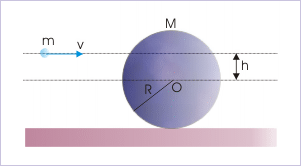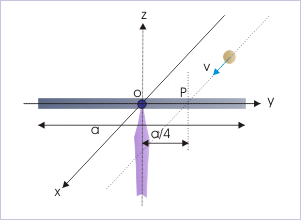# 20.6 Conservation of angular momentum (application)  (Page 6/6)

 Page 6 / 6

Problem : A solid sphere of mass 1 kg and radius 10 cm lies on a horizontal surface. A particle of mass 0.01 kg moving with velocity 10 m/s parallel to horizontal surface hits the sphere at a vertical height 6 cm above the center of the sphere and sticks to it. Find (i) the angular velocity of the sphere just after the collision and (ii) the translation velocity of the sphere, if its motion is pure rolling after collision.A particle, moving parallel to horizontal surface, strikes the sphere at a vertical elevation “h” with respect to center.

Solution : First, we need to check for external torque to ensure that there is no external torque on the system. Here, the disk and particle are acted by gravity. The force of gravity on the disk passes through its COM and does not constitute torque. The force of gravity on the particle, however, constitutes torque about the axis of rotation, but question neglects this torque as particle in moving horizontally. As such, we can proceed to apply conservation of angular momentum in the direction of axis of rotation, using scalar component form. Let “P”, “S” and “C” denote particle, sphere and combined mass respectively, then :

$\begin{array}{l}{L}_{i}={L}_{f}\\ ⇒{L}_{\mathrm{Pi}}+{L}_{\mathrm{Si}}={L}_{\mathrm{Cf}}\end{array}$

Let us consider that "m" and "M" be the masses of particle and sphere respectively and "R" be the radius of the sphere. Let us also consider that the vertical elevation of the point above center is "h". Now, the initial angular momentum of the particle is :

$\begin{array}{l}{L}_{\mathrm{Pi}}=mv{r}_{\perp }=-mvh\end{array}$

Negative sign shows that the angular momentum of the particle is perpendicular and into the plane of the disk (clockwise). The initial angular momentum of the sphere ( ${L}_{\mathrm{Si}}$ ) is zero.

Subsequent to collision, the moment of inertia of the combined mass (disk and particle) after collision is :

$\begin{array}{l}{I}_{C}=\frac{2M{R}^{2}}{5}+m{R}^{2}\end{array}$

We should note that though particle sticks at a vertical height “h” above COM, but its distance from COM is “R”. For this reason, its MI about COM involves “R” – not “h”.

Let the angular velocity of the composite system of sphere and the particle be “ω”. Now, putting values in the equation of conservation of angular momentum, we have :

$\begin{array}{l}-mvh+0={I}_{C}\omega =\left(\frac{2M{R}^{2}}{5}+m{R}^{2}\right)\omega \end{array}$

$\begin{array}{l}⇒\omega =-\frac{mvh}{\left(\frac{2M{R}^{2}}{5}+m{R}^{2}\right)}\end{array}$

Putting numerical values,

$\begin{array}{l}⇒\omega =-\frac{0.01\phantom{\rule{2pt}{0ex}}x\phantom{\rule{2pt}{0ex}}10\phantom{\rule{2pt}{0ex}}x\phantom{\rule{2pt}{0ex}}0.06}{\left(\frac{2\phantom{\rule{2pt}{0ex}}x\phantom{\rule{2pt}{0ex}}1\phantom{\rule{2pt}{0ex}}x\phantom{\rule{2pt}{0ex}}{0.1}^{2}}{5}+0.01\phantom{\rule{2pt}{0ex}}x\phantom{\rule{2pt}{0ex}}{0.1}^{2}\right)}=-1.463\phantom{\rule{2pt}{0ex}}\mathrm{rad}/s\end{array}$

The negative sign of angular velocity shows that sphere along with stuck particle rotates in clockwise direction. According to the second part of the question, the motion of the combined mass is pure rolling. Hence, translational velocity after collision is :

$\begin{array}{l}v=\omega R=1.463\phantom{\rule{2pt}{0ex}}x\phantom{\rule{2pt}{0ex}}0.1=0.1463\phantom{\rule{2pt}{0ex}}m/s\end{array}$

Example 6

Problem : A rod of mass "M" and length, "a", is pivoted at middle point "O" such that it can rotate in horizontal plane. A mud ball of mass "M/12", moving in horizontal plane strikes the rod at the point "P" with a speed "v" as shown in the figure. If the mud ball sticks to the rod after collision, find the magnitude of the angular velocity of the rod immediately after the ball strikes the rod.A mud ball, moving in horizontal plane strikes the rod at the point "P" with a speed "v".

Solution : We first need to determine whether there is any external torque on the system of rod and mud ball. The forces during collision constitute internal torques. On the other hand, the force at the pivot passes through the axis of rotation. It has no moment arm about it and as such, it does not constitute a torque. The gravitation pull on the mud ball is in vertical direction. This force constitute a torque in "-x"-direction (apply right hand rule to assess the direction). We can, therefore, conclude that there is no component of external torque in z -direction on the system before, during and after the collision.

We observe here that the rod is capable to rotate in the horizontal plane. Also, the motion of the mud ball is in the horizontal plane. The directions of angular momentums for both rod and the mud ball are along vertical direction (z-direction). We can, therefore, apply conservation of angular momentums along the direction of axis of rotation i.e. perpendicular to the planes of motion. This enables us to use the component form of conservation law in z-direction as scalar expression with only two directions. Let “R” and “B” subscripts denote rod and the mud ball respectively, then :

$\begin{array}{l}{L}_{i}={L}_{f}\\ ⇒{L}_{\mathrm{Ri}}+{L}_{\mathrm{Bi}}={L}_{\mathrm{Rf}}+{L}_{\mathrm{Bf}}\end{array}$

Here, the initial angular momentum of the rod is zero as it is stationary. Initial angular momentum of the mud ball just before it strikes the rod is given by,

$\begin{array}{l}{L}_{\mathrm{Bi}}=-m{r}_{\perp }v=-\frac{Mva}{12x4}\end{array}$

Note in the above equation that mass of the mud ball is M/12 and its moment arm is "a/4". Negative sign to show that initial angular momentum of the mud ball is clockwise.

Since the mud ball sticks to the rod, two entities become one and move with a common angular velocity. Let us now consider their common angular velocity is "ω". The final angular momentum of the rod is given by :

$\begin{array}{l}{L}_{\mathrm{Rf}}={I}_{R}\omega =\frac{M{a}^{2}\omega }{12}\end{array}$

The angular momentum of the mud ball as part of the rotating rod is given by :

$\begin{array}{l}{L}_{\mathrm{Bf}}=\frac{M}{12}x{\left(\frac{a}{4}\right)}^{2}\end{array}$

Putting these values in equation of conservation of angular momentum, we have :

$\begin{array}{l}0-\frac{Mva}{12x4}=\left(\frac{M{a}^{2}}{12}+\frac{M}{12}x\frac{{a}^{2}}{16}\right\}\omega =\frac{17M{a}^{2}\omega }{12x16}\end{array}$

$\begin{array}{l}\omega =-\frac{12x16Mva}{48x17M{a}^{2}}=-\frac{4v}{17a}\end{array}$

Thus, magnitude of angular velocity of the mud ball just after the collision is :

$\begin{array}{l}\omega =\frac{4v}{17a}\end{array}$

List the application of projectile
pls explain what is dimension of 1in length and -1 in time ,what's is there difference between them
what are scalars
show that 1w= 10^7ergs^-1
what's lamin's theorems and it's mathematics representative
if the wavelength is double,what is the frequency of the wave
What are the system of units
A stone propelled from a catapult with a speed of 50ms-1 attains a height of 100m. Calculate the time of flight, calculate the angle of projection, calculate the range attained
58asagravitasnal firce
Amar
water boil at 100 and why
what is upper limit of speed
what temperature is 0 k
Riya
0k is the lower limit of the themordynamic scale which is equalt to -273 In celcius scale
Mustapha
How MKS system is the subset of SI system?
which colour has the shortest wavelength in the white light spectrum
if x=a-b, a=5.8cm b=3.22 cm find percentage error in x
x=5.8-3.22 x=2.58

#### Get Jobilize Job Search Mobile App in your pocket Now!By Prateek AshtikarBy Alec MoffitBy Yasser IbrahimBy Mucho MizindukoBy OpenStaxBy OpenStaxBy OpenStaxBy OpenStaxBy Michael PittBy Mary Matera